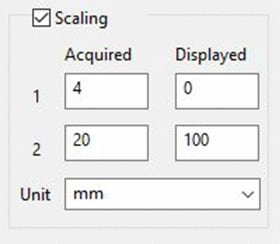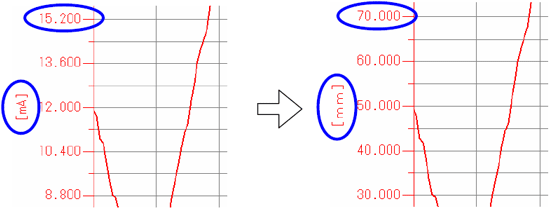# How to Convert Current Values to the Actual Measured Values of a Sensor

There are various types of sensors equipped with 4 to 20 mA output, such as displacement sensors, pressure sensors, and flow sensors. This section explains how to make it easier to understand measurement results from a sensor equipped with 4 to 20 mA output as current values.

## Scaling of measured values

You can make measurement results easier to understand by converting measured current values to the actual measured values of a sensor and adding a unit of measurement. The scaling function of an instrument is used for conversion to actual measured values. The scaling function is set through 2-point correction using two arbitrary points.

Scaling settings4 mA is scaled to 0, and 20 mA is scaled to 100.
The unit is converted to “mm.”
When current is converted to voltage using a shunt resistor, voltage values are scaled to actual measured values.

Results of scaling (measured values and units before and after scaling)INDEX